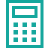### ASTM D4169 Vehicle Stacking / Stacked Vibration Top Load

\begin{array}{l}L=M_{f} \times J \frac{l \times w \times h}{K} \times \frac{H-h}{h} \times F \\{\text {Where: }} \\ {\text {L = computed top load, lbf }} \\ {\text {Mf = shipping density factor, lb/ts }} \\ {\mathrm{J}=1 \mathrm{lbf} / \mathrm{lb} \text { (conversion factor) }} \\ {\mathrm{H}=\text { max height of stack in vehicle, in }} \\ {\mathrm{h}=1 \text { eight of shipping unit or individual container, in }} \\ {\mathrm{K}=1728 \mathrm{in} 3 / \mathrm{ft} 3} \\ {\mathrm{F}=\text { combined effect factor (use } \\10 \\ \text { for ALI, } \\7\\ \text { for ALII, } \\5\\ \text { for ALIII) }}\end{array}ASTM D4169 Vehicle Stacking / Stacked Vibration Top Load Calculator

Invalid Input

Inches

Invalid Input

Inches

Invalid Input

Inches

Invalid Input

Inches

Invalid Input

Invalid Input

Calculate
Thanks for using our services!
Enter some brief information to see results
• We will send you newsletters that you can opt-out for at anytime. We do not share your information.
See ResultsASTM D4169 Vehicle Stacking / Stacked Vibration Top Load CalculatorLength (l):
Width (w):
Height (h):
Maximum Stack Height in Vehicle (H):
Shipping Density (Mf):
F Factor:
Reset
NOTES
1. This formula is for Vehicle Stacking made up of mixed commodities shipped in a Less-Than-Truck-Load (LTL) or small package delivery environment.
2. Maximum Stack Height (H) is 54 inches or 108 inches per the standard.
3. Shipping Density (Mf) is almost always 10, per the standard
4. The results of the Computed Top Load formula can be used as reference however must not be used to rate the ability of shipping containers to withstand an actual top load. Such an estimate shall not be used in place of testing conditioned test samples in a controlled, repeatable, test environment with calibrated instrumentation, validated machines, and trained test operators.ASTM D4169 Vehicle Stacking / Stacked Vibration Top Load Calculator

Invalid Input

Inches

Invalid Input

Inches

Invalid Input

Inches

Invalid Input

Inches

Invalid Input

Invalid Input

Calculate
Thanks for using our services!
Enter some brief information to see results
• We will send you newsletters that you can opt-out for at anytime. We do not share your information.
See ResultsASTM D4169 Vehicle Stacking / Stacked Vibration Top Load CalculatorLength (l):
Width (w):
Height (h):
Maximum Stack Height in Vehicle (H):
Shipping Density (Mf):
F Factor: